### [求助]NET编译的要怎么破解

vagary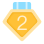2013-12-25 23:43
10423

[2023春季班]《安卓高级研修班(网课)》月薪两万班招生中～chixiaojie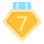2013-12-25 23:52
2
0
.net reflector 配上用场了。vagary2013-12-26 00:03
3
0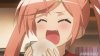heidongqi2013-12-26 10:14
4
0
http://blog.csdn.net/u010561359/article/details/8986660liuyilin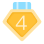2013-12-26 10:25
5
0prize2013-12-26 15:03
6
0
reflector + Reflexil插件：静态分析+程式修改

ilspy：静态分析+动态调试

de4dot：反混淆，包括很多主流的保护方式vagary2013-12-26 16:34
7
0vagary2013-12-26 16:35
8
0vagary2013-12-31 09:30
9
0luyikk2013-12-31 21:22
10
0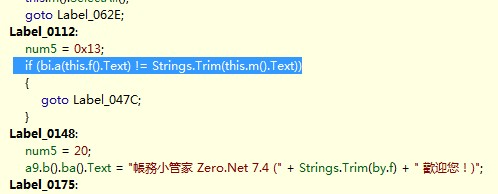找到比对的方法 原来是通过 EMAIL +一个算法生产的序列号。这里是 反运算一次，然后和EMAIL 进行比对如果OK 那么 去  http://www.mysoft.idv.tw/mymoneymail.txt 查找有没有这个邮箱

```        static string a(string A_0)
{

string str5 = "";

StringType.MidStmtStr(ref A_0, 1, 1, " ");

StringType.MidStmtStr(ref A_0, 2, 1, " ");

StringType.MidStmtStr(ref A_0, 3, 1, " ");

A_0 = A_0.Trim();

if ((A_0.Length % 5) == 0)
{
if (A_0.Length >= 20)
{
if (A_0.Trim() != "")
{
int num8 = A_0.Length;
int start = 1;

Re:
if (start <= num8)
{
string str = Strings.Mid(A_0, start, 5);

int num4 = Strings.Asc(Strings.Mid(str, 1, 1));

int num5 = Strings.Asc(Strings.Mid(str, 4, 1));

string str2 = Strings.Mid(str, 2, 1);

string str3 = Strings.Mid(str, 3, 1);

string str4 = Strings.Mid(str, 5, 1);

int num6 = 0;

if (num4 <= num5)
{
num6 = num5 - num4;
}

num6 = num4 - num5;

int num = (Strings.Asc(str2) - num6) - 0x41;

int num2 = (Strings.Asc(str3) - num6) - 0x41;

int num3 = (Strings.Asc(str4) - num6) - 0x41;

string am1 = Conversions.ToString(num);
string am2 = Conversions.ToString(num2);
string am3 = Conversions.ToString(num3);
double zm = Conversion.Val( am1+am2+am3);

str5 = str5 + Conversions.ToString(Strings.Chr((int)Math.Round(zm)));

start += 5;

goto Re;
}
}

}
}

return str5;

}
```

```        static string DE(string a)
{
string re = "";

foreach (char c in a)
{
int m = Strings.Asc(c);

int am1 = 51;
int am2 = 50;

string zm = m.ToString();

int x1 = 0;
int x2 = 0;
int x3 = 0;

x1 = 0;
x2 = Convert.ToInt32(zm.ToString());
x3 = Convert.ToInt32(zm.ToString());

x1 += 1;
x2 += 1;
x3 += 1;
x1 += 0x41;
x2 += 0x41;
x3 += 0x41;

string mr = "";
mr += Strings.Chr(am1);
mr += Strings.Chr(x1);
mr += Strings.Chr(x2);
mr += Strings.Chr(am2);
mr += Strings.Chr(x3);

re += mr;
}

re = "abc" + re;

int mxxx = re.Length;

return re;
}```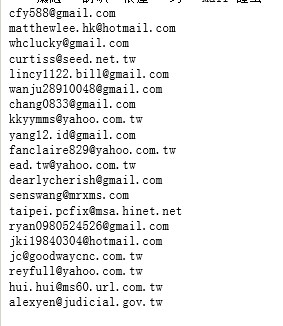luyikk2013-12-31 21:27
11
0luyikk2013-12-31 21:29
12
0vagary2014-1-2 08:07
13
0
@.@~~~vagary2014-1-2 08:10
14
0luyikk2014-1-2 19:18
15
0
.NET 不需要调试，看看代码就知道他干嘛了。 对付JAVA 和.NET 我一般都不调试的。vagary2014-1-2 21:32
16
0donpps2014-1-4 17:13
17
0

```        static string MMZ_RegCode(string email)
{
string regcode = "";
Random random = new Random(); //随机种子
foreach (char email_char in email)
{
//电子邮箱的每个字符演算成5个字符组成注册码的主体部分。
string email_char_ascii = string.Format("{0:D3}", (UInt16)email_char); //电子邮箱字符串的每个字符取ASCII值，格式化成3位10进制数值;

int regcodepart_1 = random.Next(65, 90); // 随机产生一个A..Z之间的随机字母，产生第一位字符的ASCII值。
int regcodepart_4 = random.Next(65, 90); // 随机产生一个A..Z之间的随机字母，产生第四位字符的ASCII值。

int regcodepart_2 = Convert.ToUInt16(email_char_ascii.ToString()); //电子邮箱字符串得到的3位10进制数值，百位。
int regcodepart_3 = Convert.ToUInt16(email_char_ascii.ToString()); //电子邮箱字符串得到的3位10进制数值，十位。
int regcodepart_5 = Convert.ToUInt16(email_char_ascii.ToString()); //电子邮箱字符串得到的3位10进制数值，个位。

regcodepart_2 += Math.Abs(regcodepart_1 - regcodepart_4); //加上第一位字符与第四位字符ASCII值的差的绝对值。
regcodepart_3 += Math.Abs(regcodepart_1 - regcodepart_4); //加上第一位字符与第四位字符ASCII值的差的绝对值。
regcodepart_5 += Math.Abs(regcodepart_1 - regcodepart_4); //加上第一位字符与第四位字符ASCII值的差的绝对值。

regcodepart_2 += 0x41; //加上65，产生第二位字符的ASCII值。
regcodepart_3 += 0x41; //加上65，产生第三位字符的ASCII值。
regcodepart_5 += 0x41; //加上65，产生第五位字符的ASCII值。

string regcodemain = "";
regcodemain += (char)regcodepart_1; // 得到第一位字符。
regcodemain += (char)regcodepart_2; // 得到第二位字符。
regcodemain += (char)regcodepart_3; // 得到第三位字符。
regcodemain += (char)regcodepart_4; // 得到第四位字符。
regcodemain += (char)regcodepart_5; // 得到第五位字符。

regcode += regcodemain; //得到主体部分。
}

regcode = "MMZ" + regcode; //前面再加上程序的简称。
return regcode;
}```vagary2014-1-6 12:43
18
0donpps2014-1-6 21:37
19
0vagary2014-1-7 12:43
20
0

EXCEL也能做出注册机YUEN2014-2-19 10:30
21
0checktt2014-5-7 17:47
22
0
.net reflector8.3